## What will you learn?

###### This post is also available to you in this video, should you be interested 😉

In our previous post, we talked about the derivative of the softmax function with respect to its input. We indeed beautifully dissect ed the math and got comfortable with it! In this post, we will go one step further! Let’s say you have a neural network with softmax output layer, and you are using the cross-entropy error function. Today, we will derive the gradient of the cross-entropy error with respect to the input of the softmax function.

This is one of the most confusing mathematical derivations that machine learning enthusiasts tend to have problems with. This post is important as it shows you, if you have a softmax output layer, and use the cross-entropy function, how you can apply back-propagation from the error all the way to the input of the softmax layer, which is the tricky part of the entire back-propagation in this type of neural networks.

## A Typical Neural Network

Let’s consider a simple neural network with D-dimensional input data, andoutput neurons with softmax output function. So, foroutput neurons, we will havesoftmax outputs. We will have one-hot encoding ground-truth vectors and finally we will have the cross-entropy error function to compute the distance between the output vector and the ground-truth vector. This is a typical neural network for a multi-class classification task. So, for a given training example as the input, the network will generate an output vector with the size of(which is the number of classes in our dataset)!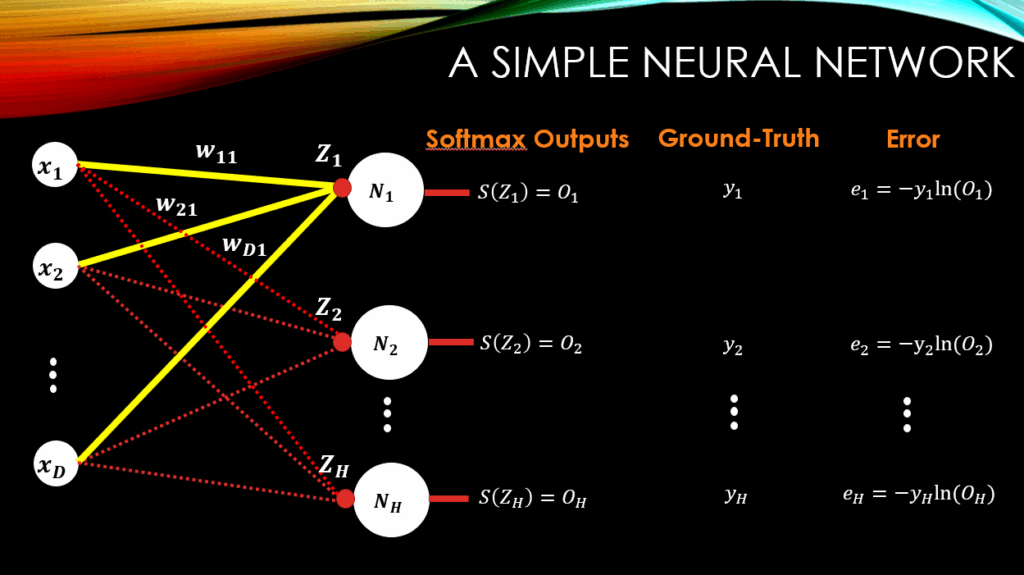So, just as a gentle reminder, the softmax function can be defined as:And the interesting property of this function is that the sum of all the outputs of softmax, is always equal to 1:Now, about the ground-truth vector,, we mentioned that it is a one-hot encoding vector. This means that for every ground-truth vector ONLY one element can be equal to 1 and all the other elements are equal to 0!

Finally, regarding the cross-entropy error function, the mathematical representation of this function is as follows:In this definition of error,, is the natural logarithm. As for an example, let’s say we have 3 output neurons. For a given training example, the output vector of this neural network will have 3 elements in it. Let’s say the output vector is as follows:You notice that these sum up to 1 as the property of softmax function. And, let’s say the ground-truth vector for the same input training example is as follows:###### With s simple comparison between the network output vector and the ground-truth vector you can see that, the network thinks that the given training example belongs to class 2 (as 0.6 is the largest value and corresponds to class 2), however, the ground-truth says that the training example actually belongs to class 1 (as only the value corresponding to the first class is 1 and all the others are 0).

Now, let’s see how we can compute the cross-entropy error function:which is equal to:which is:Now that we are comfortable with the whole setting, let’s see how we can derive the gradient of this error function with respect to the inputs of the softmax output function and apply back-propagation from scratch!

## Deriving Back-propagation through Cross-Entropy and Softmax

###### In order to fully understand the back-propagation in here, we need to understand a few mathematical rules regarding partial derivatives.

Rule 1) Derivative of a SUM is equal to the SUM of derivatives

Rule 2) The rule of IndependenceRule 3) The Chain Rule

ifis a function of(i.e.,) andis a function of(i.e.,) then:Rule 4) Derivative of the log function###### Now, in order to apply back-propagation, we will have to compute the gradients of the total error w.r.t the inputs of the softmax layer. Please note that this is only the beginning of the back-propagation! If you had 20 layers in your neural network, you would have to compute the gradient of your error w.r.t all the learnable parameters across all 20 layers! However, I believe this initial part is the step where most people are not quite comfortable with. Now, let’s see how we can compute the gradient of the total error w.r.t the inputs of the softmax layer: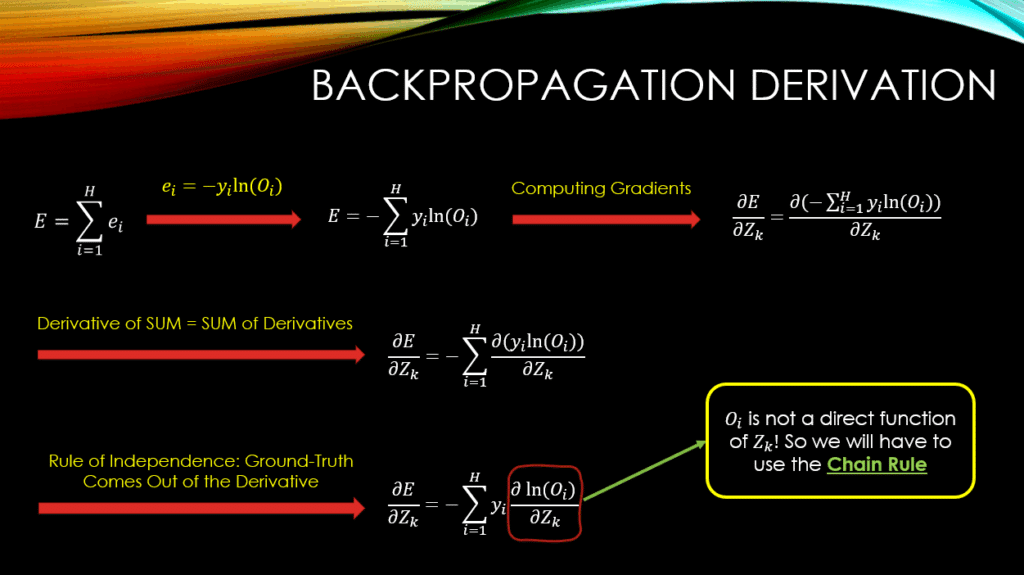###### Why the chain rule? Well because in, we notice thatis not a direct function of! However, it is a direct function of! In turn,is indeed a direct function of! So, in order to compute, we will have to go throughfirst and then get to! By chaining these steps together we will have our chain!!!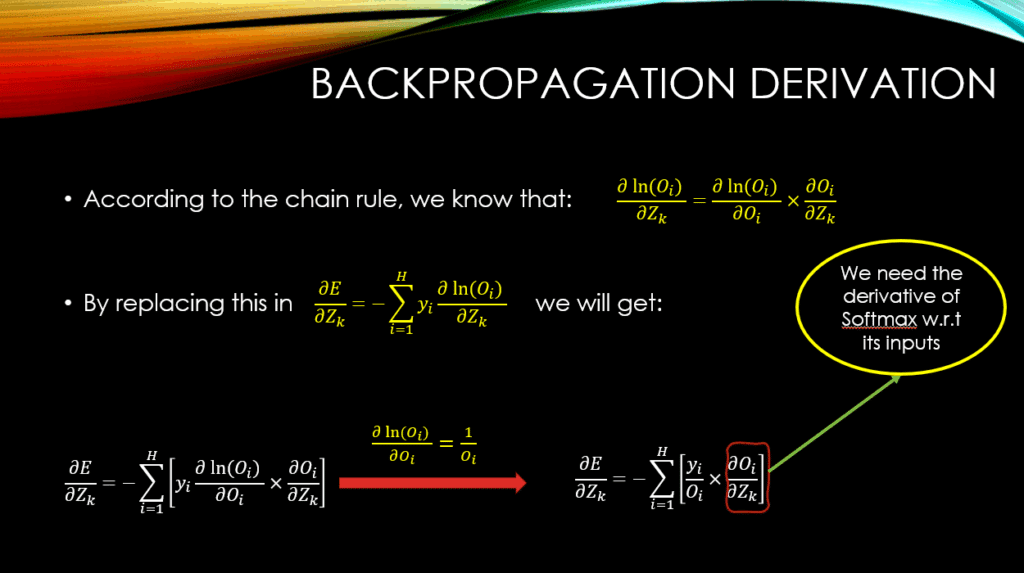Now after applying the chain rule, you notice that we have also used Rule #4 to find the derivative of our natural logarithm, that is,. We have got to the place where we will need the derivative of the softmax function w.r.t its input (i.e.,). We have already covered the derivative of softmax w.r.t its input in our previous post, but as a reminder, see the slide blow: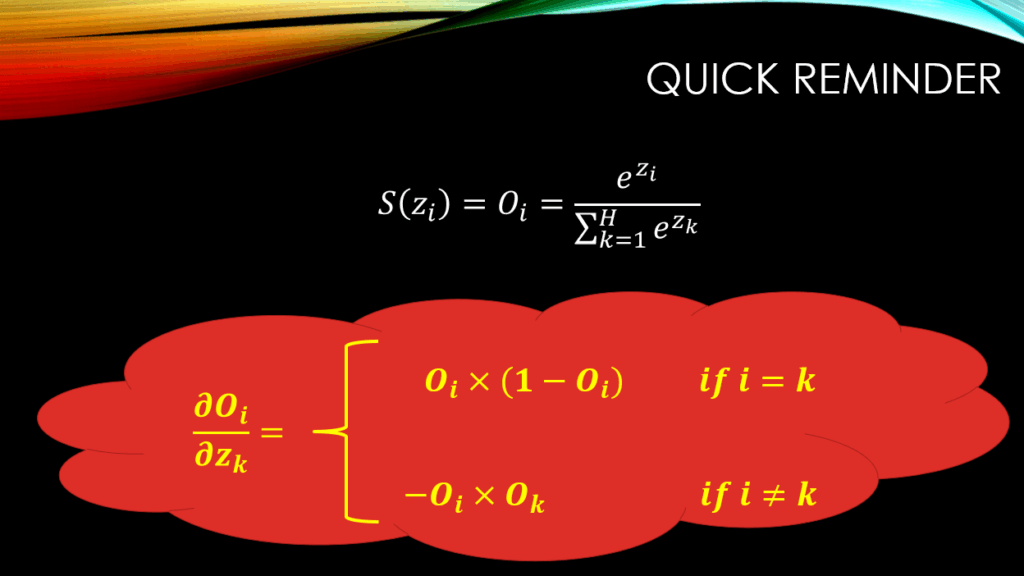###### So, we can see that when it comes to, it all boils down to whetheror! As a result, in order to compute, for a given, we will consider all‘s for! There will be ONLY one case where, and for all other values of, for sure we will have! Thus let’s  solvefor both cases: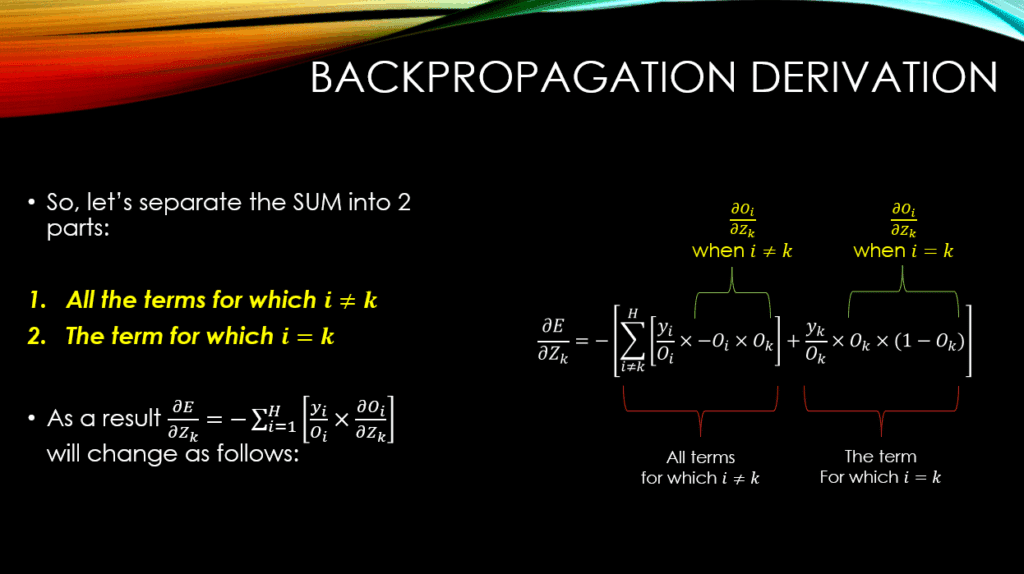Now, the hard part is over! You can see how we have taken out  the term for which! Then we have used the derivative of softmax (extensively discussed in our previous post) in order to finally be done with the partial derivative operations. Now, let’s simplify: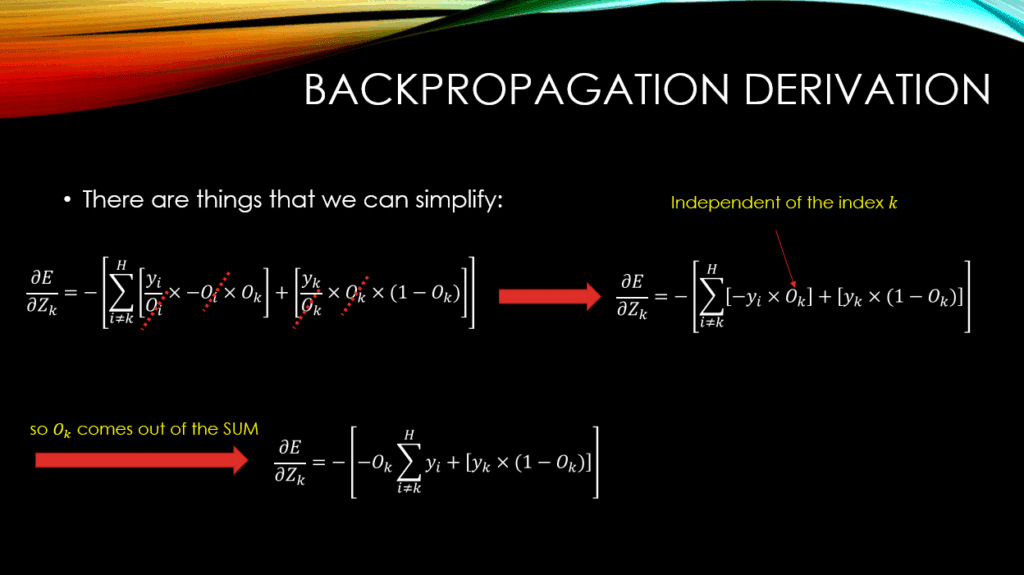###### In order to get rid of the summing operation, the next trick we can play is taking advantage of the fact that, the ground-truth vector, is a one-hot encoding vector! As a result, if we sum all of its elements across alloutputs, the result will always be equal to 1 (i.e.,). So, in order  to compute, we can subtract theelement of(i.e.,), from. This is how we will get rid of the SUM from our math! See below: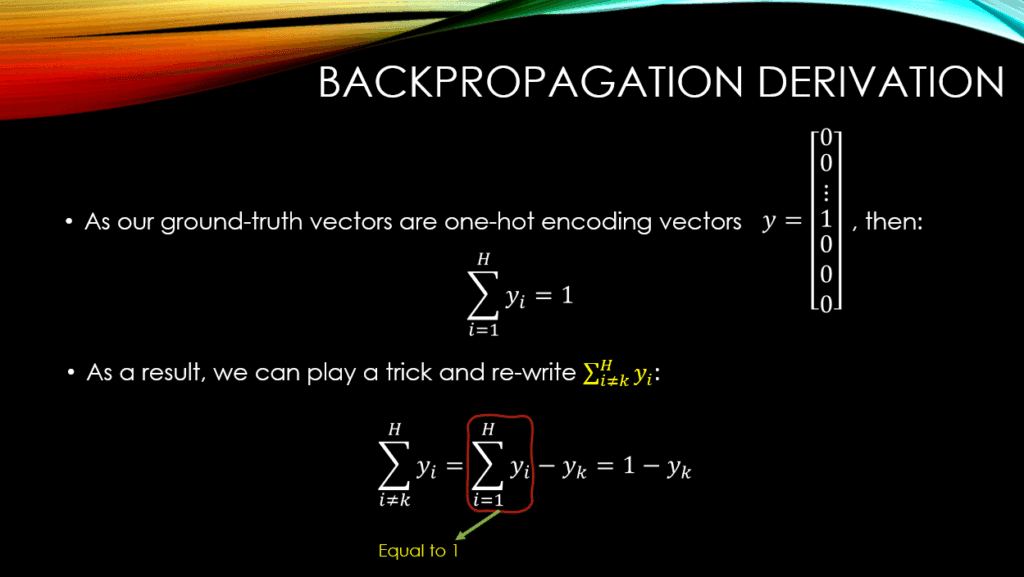###### So, now that we have computedwith our smart approach, let’s replace it in our derivations, and simplify more: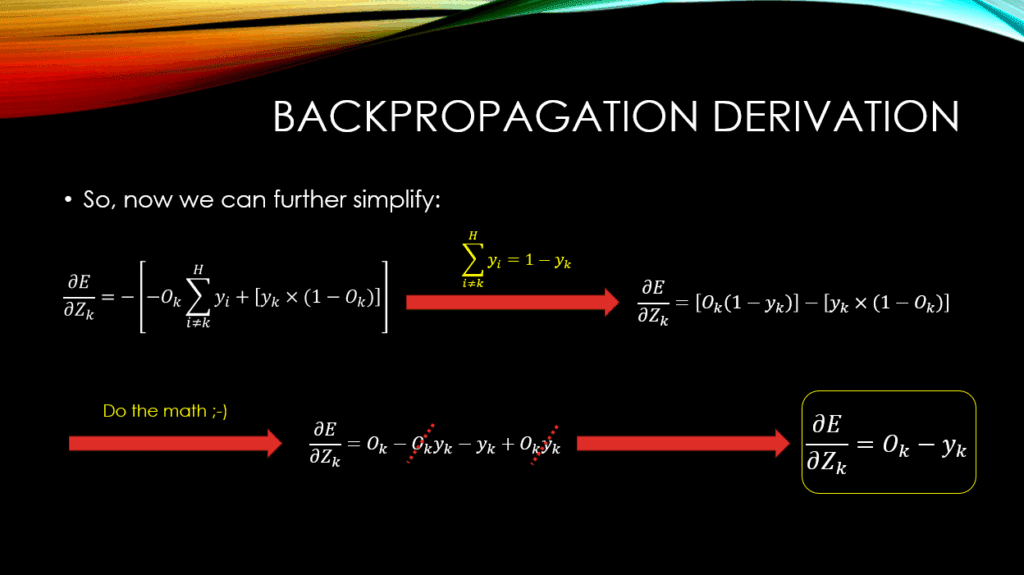###### Perfect! We are finally done! Let’s see the final answer in a nice and concise way: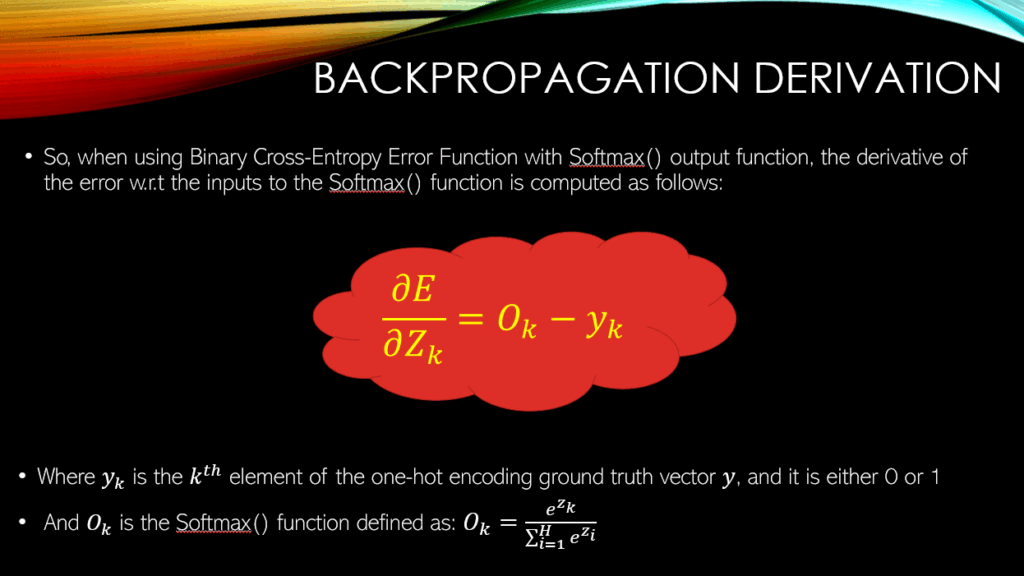## Conclusions

So there you have it! Now you can see the very simple outcome of this whole monstrous series of derivations! The fact that it could be simplified to this level, is the benefit of using the cross-entropy error function with softmax output function in neural networks! However, this simplicity is not the main reason as to why we use softmax coupled with cross-entropy error function, mind you! This is just the nice outcome that we can enjoy!

Until next time, on behalf of MLDawn

Take care 😉